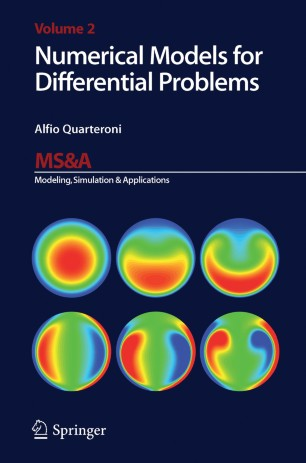# Numerical Models for Differential Problems

• Alfio QuarteroniTextbook

Part of the MS&A book series (MS&A, volume 2)

1. Front Matter
Pages I-XVI
2. Pages 11-29
3. Pages 31-59
4. Pages 119-138
5. Pages 139-157
6. Pages 159-172
7. Pages 173-216
8. Pages 217-226
9. Pages 227-269
10. Pages 271-312
11. Pages 313-344
12. Pages 383-400
13. Pages 401-455
14. Pages 457-500
15. Pages 501-546
16. Back Matter
Pages 581-601

### Introduction

In this text, we introduce the basic concepts for the numerical modelling of partial  differential equations. We consider the classical elliptic, parabolic and hyperbolic linear equations, but also the diffusion, transport, and Navier-Stokes equations, as well as equations representing conservation laws, saddle-point problems and optimal control problems. Furthermore, we provide numerous physical examples which underline such equations. We then analyze numerical solution methods based on finite elements, finite differences, finite volumes, spectral methods and domain decomposition methods, and reduced basis methods.

In particular, we discuss the algorithmic and computer implementation aspects and provide a number of easy-to-use programs.

The text does not require any previous advanced mathematical knowledge of partial differential equations: the absolutely essential concepts are reported in a preliminary chapter. It is therefore suitable for students of bachelor and master courses in scientific disciplines, and recommendable to those researchers in the academic and extra-academic domain who want to approach this interesting branch of applied mathematics.

### Keywords

Analysis Numerical modelling PDE algorithms finite element method hyperbolic equation partial differential equation

#### Authors and affiliations

• Alfio Quarteroni
• 1
• 2
1. 1.MOX, Department of Mathematics “F. Brioschi”Politecnico di MilanoMilanItaly
2. 2.CMCS-IACSEcole Polytechnique Fédérale de LausanneLausanneSwitzerland